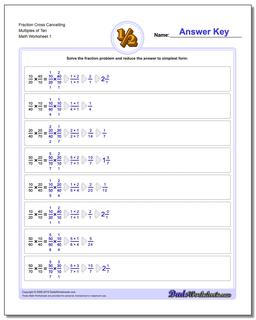# Math Worksheets: Fraction Multiplication: Fraction Multiplication: Fraction Cross Cancelling Multiples of Ten## Fraction Cross Cancelling Multiples of Ten

PropertyValue
DescriptionFraction Cross Cancelling Multiples of Ten: The multiplying fraction worksheets in this section have a heavy emphasis on problems that require you to cross multiply (or cross cancel) to get the product.
Resource TypeWorksheet